This page has been robot translated, sorry for typos if any. Original content here.

 Section Start Industrial, amateur Amateur Radio Aircraft model, rocket model Useful, entertaining Tricks to the master Electronics Physics Technologies Inventions Secrets of the cosmos Secrets of the Earth Secrets of the Ocean Tricks Section map Use of site materials is permitted subject to a link (for sites - hyperlinks)
 Navigation: => Home / Physics / Research /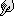HYPOTHESIS OF ABSOLUTE SPACE (alternative hypothesis of gravity)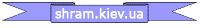Cherednichenko V.I.

The ABSOLUTE SPACE hypothesis can be formulated as follows: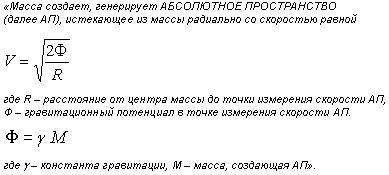The speed of AP at any point in the Universe is equal to the vector sum of the velocities from each of the masses of the Universe. On Earth, the AP speed from all the masses of the Universe, except the Solar system, is close to zero, since the AP speeds from each mass cancel each other out. That is, on Earth, it is possible to take into account mainly the AP speeds created by the Sun and the planets of the solar system. Having calculated the speed of the center of the Earth relative to the AP, we can assume that, according to the law of inertia, this speed remains constant until the Earth collides with any object. On the surface of the Earth, the AP speed is 11.2 km / s.

All masses (bodies) of the Universe have a constant speed relative to AP. Bodies are not attracted to each other, in the AP hypothesis there is generally no need to introduce gravitational forces. The motion of all the masses of the universe occurs as a result of inertia. Since in AP everything moves by inertia and there are no accelerations, then AP is an absolute inertial reference system. But this is not Newton’s motionless space: each AP point moves relative to distant stars in accordance with the gravitational potential at a given point. Because Since potential is not absolute, then we can only operate with the potential difference. Accordingly, you can attach any speed to the AP speed, but by determining the value of the AP speed at a given point, we thereby fix the speeds of all AP points relative to this point.

Since the values ​​of the constants from the Newtonian hypothesis are used in the AP hypothesis, all experimental results are the same as Newton’s, the picture of the motion of the bodies of the Universe corresponds to the picture drawn by Newton, it is impossible to experimentally refute the AP hypothesis (using already conducted experiments), but it is simpler and more concise in nature: there is no need to introduce such concepts as long-range, force field, and heavy mass to explain the observed phenomena. In fact, the AP hypothesis offers just a different interpretation of the observed facts, allowing according to the principle of "Occam's razor" to cut off "entities invented beyond what is necessary." But at the same time, the AP hypothesis is not speculative; it satisfies the criteria of K. Opera's verification and falsification: to test the AP hypothesis, one needs to compare light speeds in the horizontal and vertical directions on a Michelson interferometer, the difference should be 11.2 km per second. If this fact is not confirmed, then the AP hypothesis is incorrect.

Using the ABSOLUTE SPACE hypothesis, one can explain:

1. equality of gravitational and inertial masses,
2. redshift and “recession of galaxies”,
3. the paradox of the "dark sky"
5. the result of the Michelson – Morley experiment,

In addition, the AP hypothesis allows us to calculate the values ​​of the following constants:

1. acceleration of gravity,
2. radius of the visible part of the universe / Hubble constant

EQUALITY OF GRAVITATIONAL AND INERTIAL MASS

It is well known that gravitational and inertial masses (on a certain scale) are equal up to the 16th sign. This means that it is impossible to distinguish between a uniform gravitational field and a uniformly moving reference frame by any experiments. Those. an observer in a closed laboratory cannot clearly determine by any experiment: whether the force acting on the mass is gravitational or inertial.

Using this fact, Einstein showed the geometric nature of a uniform gravitational field. But for an inhomogeneous, for example, centrally symmetric gravitational field created by the mass, Einstein failed to construct an adequate reference frame and had to construct a curved space.

The AP hypothesis confirms the geometric nature of gravity and claims that the gravitational mass does not exist at all, there is only an inertial mass. The absolute space formed by the radial velocity vector field, as shown above, is used as a reference frame. The velocity vector (flow, outflow) of absolute space at any point in the AP is equal to the vector sum of the AP velocities from each mass of the Universe.

Thus, the equality of gravitational and inertial masses is not equality, but identity: in reality, physically, there is only an inertial mass, and gravitational mass is an appearance, “appearances” such as “caloric” or “phlogiston”. This is another myth of physicists, which was created something like this. All bodies (masses) either lie or hang on supports. The reactions of the supports make the bodies move accelerated (with an acceleration of 9.8 m / s2) relative to the "Absolute Space". If you remove the support, then the reaction of the support will not act on the body and the body will begin to move by inertia, i.e. body speed will be constant relative to AP. In AP, all bodies (masses) move by inertia: there are no accelerations, there are no attractive forces (nothing is attracted to anything). Thus, Newton took the reaction of the support for the force of gravity, and then it was called gravity: from here came the gravitational (otherwise called “heavy”) mass.

RED DISPLACEMENT AND CALCULATION OF THE HUBBL CONSTANT, “RUNNING OF GALAXIES”

Take a sphere with a radius of 1 megaparsec. In the center of the sphere is an observer, on the surface of the sphere is a light source. In the center of the sphere, we take the AP velocity (VAP) equal to zero (Ф = 0). On the surface of a sphere, the AP velocity is equal to: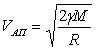Where R is the distance from the observer to the light source (radius of the sphere), M is the mass inside the sphere that creates the AP.

Substituting instead of mass the volume of the sphere multiplied by the density (4/3 * 3.14 * R3), reducing R and taking it out from under the root, we get: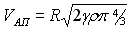Thus, we obtained that the AP speed is proportional to R, which corresponds to the Hubble law.

If we take R = 1 megaparsec, then VAP will be equal to 70.4 km / s on the surface of a sphere with a radius of 1 megaparsec. If a photon is emitted from the surface towards the center, then its speed relative to the AP is C – V (C-70.4 km / s) and this speed relative to the AP is always and everywhere. At this speed, the photon flies to the center of the sphere to the observer. In the center of the sphere, VAP is equal to zero, i.e. the observer is stationary relative to the AP and the speed of the photon relative to the observer С-V (C-70.4 km / s).

T.O. the observer will see the photon arriving from a distance of 1 megaparsec reddened and decide that the light source is moving away from it at a speed of 70.4 km / s. He will calculate the distance and note with satisfaction that the Hubble constant is within acceptable limits (at a density of 10–25 kg / m 3 ).

This is the Hubble constant. We have a choice: from the accepted density value we calculate the value of the Hubble constant, or from the measured Hubble constant we calculate the average density of matter in the Universe.

As you can see, the redshift and the value of the Hubble constant are explained by the properties of the AP without the involvement of the Big Bang and the “recession of galaxies”. According to the AP hypothesis, there was no big bang, the Universe is relatively stationary; accordingly, it is not necessary to come up with an explanation for the fact that the Earth is older than the age allotted to it by Hubble (14 billion years). Earth may be older than 100 billion years.

PARADOX OF "DARK SKY"

The paradox of the dark sky is easily explained within the framework of the AP hypothesis. As in the previous paragraph, we take a sphere of radius R of such a size that it would equal C (the speed of light) on the surface of the sphere VAP. From the same formula under the indicated condition we find R, this will be the maximum distance from which light reaches the observer, although it is very reddened. (Because the speed of light relative to AP is

С – VАП = C – C = 0, i.e. light will reach the observer at a speed equal to zero - “super reddened.”)

This sphere is the part of the Universe that we see, we will never see the rest under any circumstances. There are not so many stars in this visible part and they cannot create a continuous bright background - therefore the sky is dark. The radius of this sphere is about 4200 megaparsec (at the above average density of matter in the Universe), and beyond this distance we will not see in any telescope.

Relic radiation (1mm to 10cm) comes to us from the border of the visible part of the Universe. These are strongly reddened quanta of the entire range of radiation: visible light travels a shorter distance, x-ray and gamma quanta - more. Thus, it is not surprising that they go everywhere and densely populate this frequency range.

MICHAELSON-MORLEY EXPERIENCE

It is explained by the fact that in the horizontal plane the speed of the AP (therefore, the speed of light) is the same in all directions, respectively, no matter how you turn the interferometer, the difference in the speed of light is zero. However, if the speed of light was measured in the horizontal and vertical directions, then according to the hypothesis of AP, a difference in the speed of light equal to 11.2 km per second should be detected. (If this is confirmed, then the AP hypothesis can be considered true for some time until results are found that refute it).

EARTH ACCELERATION CALCULATION

This constant can be calculated by differentiating the speed of AP on the Earth’s surface in time.

It should be noted that the concept of ABSOLUTE SPACE in one form or another has already been put forward by some scientists, although in the presented form it has been developed and formulated by the author of the article.

Tiguntsev has a similar hypothesis, only the speed of 11.2 km / s refers to the ether flowing into the Earth, but the introduction of such a thing as ether with its hardness, elasticity, etc. leads to a number of problems. The AP hypothesis assumes that there is an empty space that has no mass, no energy, no elasticity, no hardness, and no material attributes are needed to create it, accordingly there is no problem where to get it and where to hide it later.

Much closer to the proposed hypothesis of the AP “absolute space” by Niels Björn, invented by Burlankov. AP speeds are the same in both hypotheses, only the directions are opposite. Gribanovsky E.K. it also claims that there is only an inert mass: “... in the falling space (I call it“ absolute space ”) there is no such thing as gravitational mass. There is only an inert mass ... ".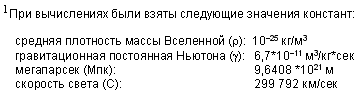print version
Author: V. Cherednichenko
PS Material is protected.
Date of publication 02.21.2007NEW ARTICLES AND PUBLICATIONSThe manufacturing technology of universal couplings for weldless, threadless, non-glossy connection of pipe sections in high pressure pipelines (video available)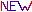Technology for refining oil and oil productsOn the possibility of moving a closed mechanical system due to internal forcesFluid glow in thin dielectric channelsThe relationship between quantum and classical mechanicsMillimeter waves in medicine. A New Look. MMB therapyMagnetic engineHeat source based on nasal aggregates